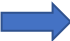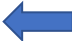## 24.2 Project phases

### The main project phases are:### After data gathering iteration is trumpFigure from (Image Credit: Owlsmcgee [Public domain] )

EDA => exploratory data analysis
source http://www.feat.engineering/intro-intro.html#the-model-versus-the-modeling-process]

• Exploratory data analysis

• Find correlations or mutial depence
• Quantiative analysis
• Check distribution
• Long tail => log of variable
• Feature engineering23
• Create and select meaningful features
• Model fit
• Selecting a few suited models
• Model tuning
• Vary model hyperpparameters

### 24.2.1 Feature engineering

Variables that go into model are called:

• Predictors• Features
• Independent variables

Quantity being modeled called:

• Prediction• Outcome
• Response
• Dependent variable

From input to output

$outcome = f(features) = f(X_1, X_2, \dots, Xp) = f(X)$

$\hat{Y} = \hat{f}(X)$

### References

———. 2018. “Feature Engineering and Selection: A Practical Approach for Predictive Models.” http://www.feat.engineering/index.html.

1. Good source for feature engineering: http://www.feat.engineering/index.html↩︎